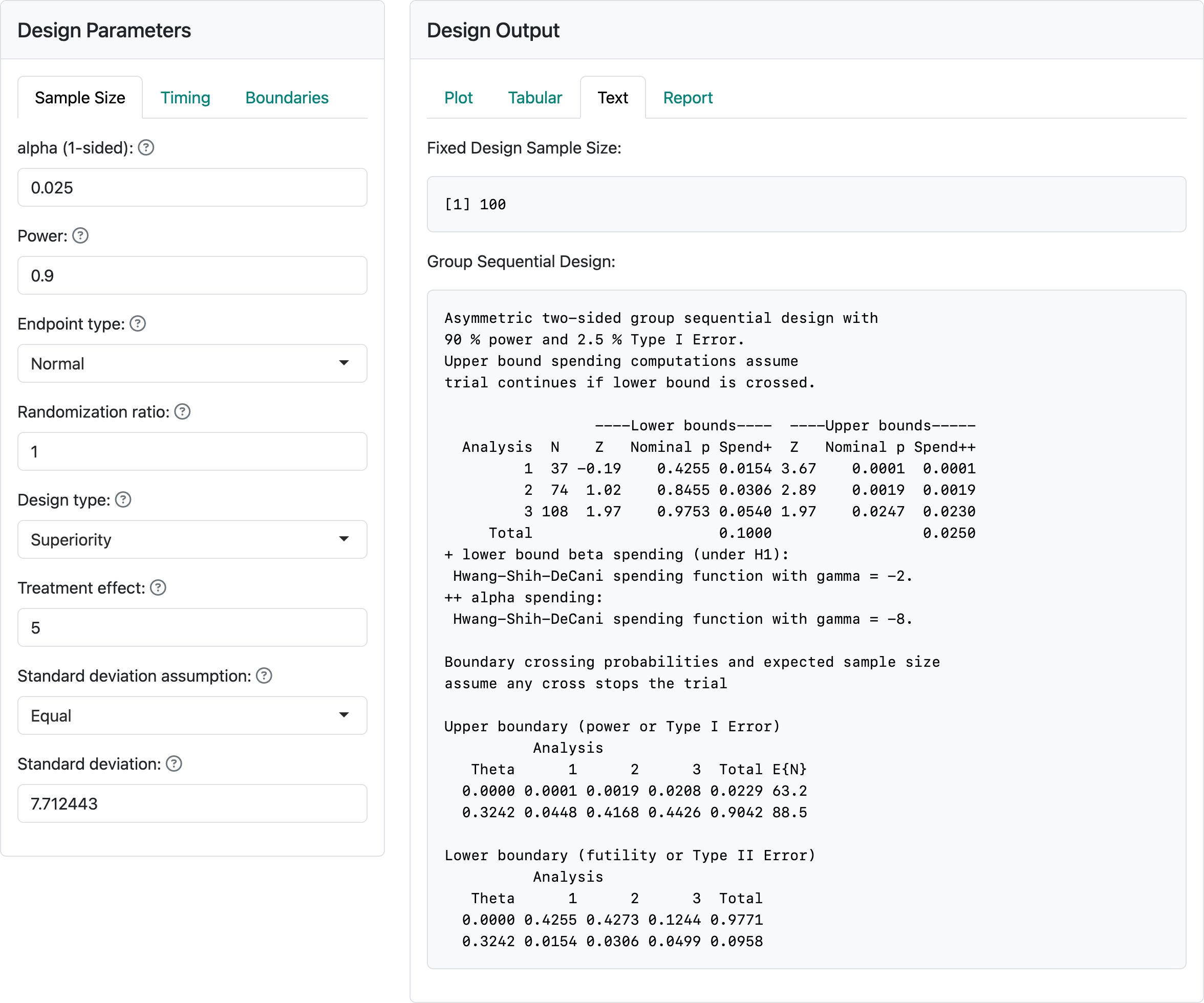# 3 Binomial and normal endpoints

We show sample size calculation when endpoints follow the binomial or normal distribution.

## 3.1 Binomial outcomes

### 3.1.1 What is a binomial outcome?

Binomial endpoints refer to outcomes where each patient either succeeds or fails at some underlying probability level. For instance, the response rate for patients to a drug is 40% or the failure rate is 15%. You would be looking for a higher response rate or a lower failure rate in the experimental arm compared to control.

The basic method for computing the fixed sample size that is the basis for group sequential design sizes for superiority was developed by Joseph L Fleiss, Alex Tytun, and Hans K Ury7, but is applied here without the continuity correction as recommended by Ian Gordon and Ray Watson8. This method was extended to noninferiority trials by Conor P Farrington and Godfrey Manning9.

### 3.1.2 Input

The graphic below shows the input values for a trial with a binomial distribution for the study outcome. The “alpha” and “Power” controls remain unchanged from the previous chapter. For the first time we see the “Randomization ratio” control which specifies the the number of experimental group patients randomized relative to control patients; this will most often be 1, specifying equal randomization between treatment groups. The next two controls specify event rates, either the success or failure rate for each treatment group.

The “H0 difference” control will normally remain as 0 as this specifies that you wish to demonstrate superiority of the experimental group compared to control. If “H0 difference” were $$< 0$$, you would be specifying a non-inferiority hypothesis, which we will discuss shortly. For the case shown here we are looking to power the trial to lower the failure rate from 15% in the control group to 10% in the experimental group.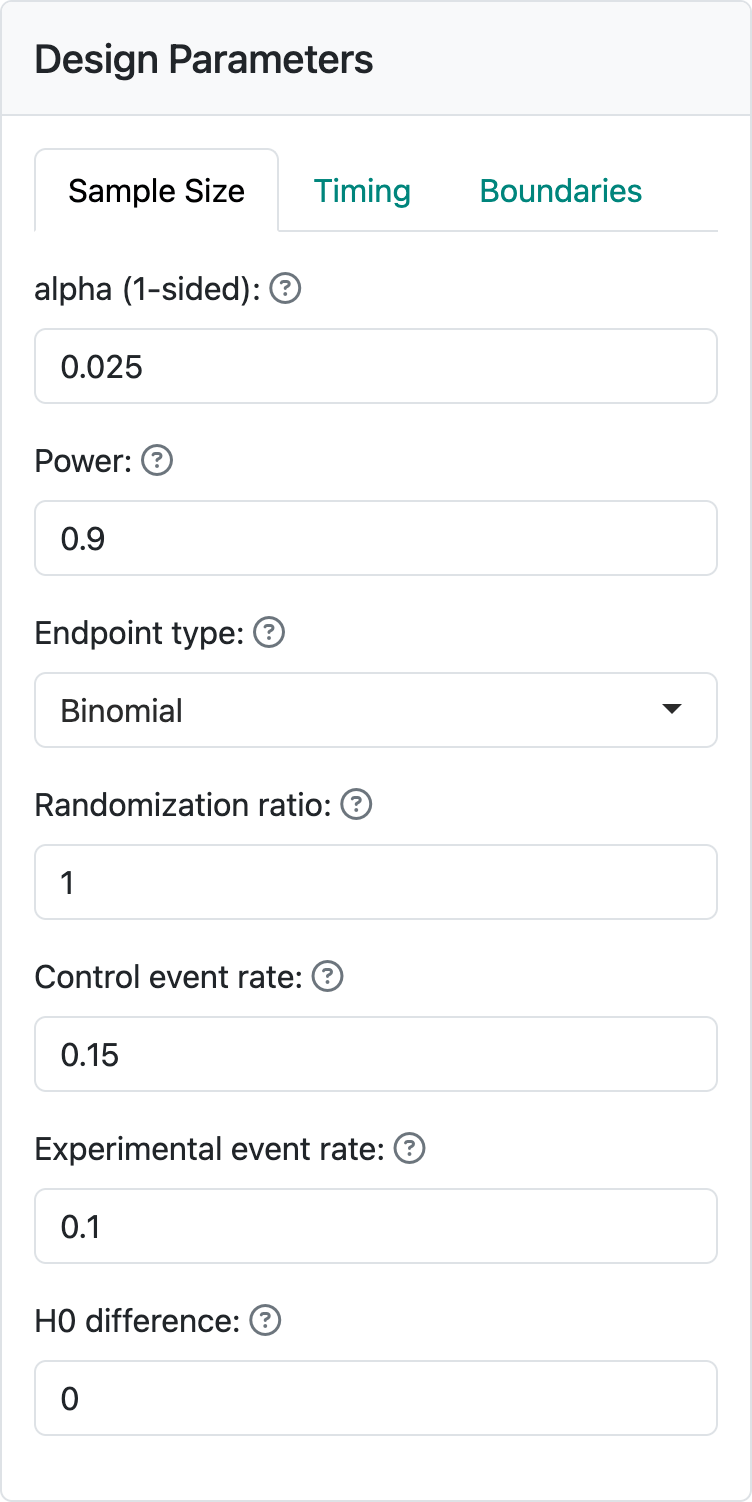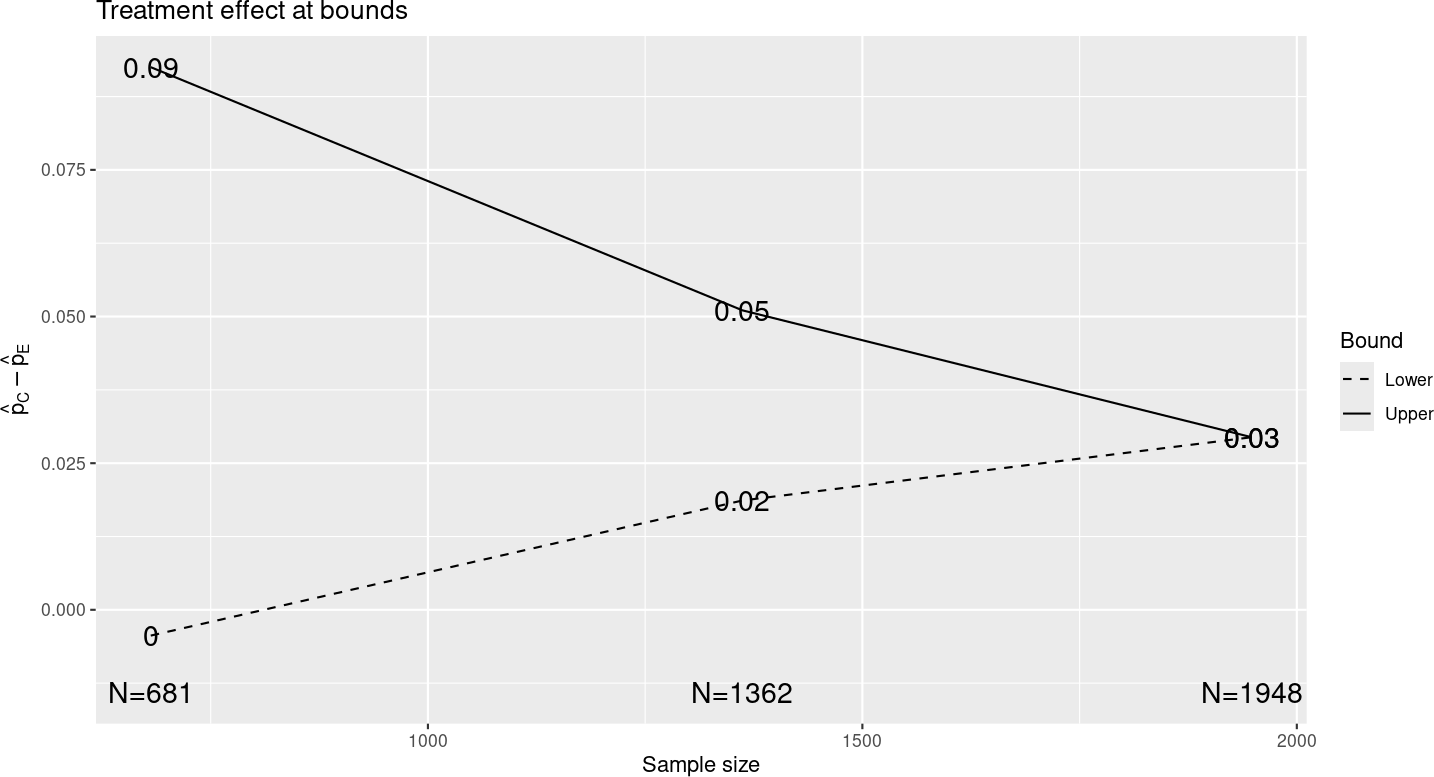### 3.1.3 Output

Assuming you have left other inputs unchanged from the previous chapter and you select the “Treatment effect” plot, you should see the plot above. This has “the same shape” as the treatment effect in the previous chapter, but the x- and y-axes have been rescaled, indicating approximate differences in observed event rates at the study bounds for a larger sample size. If you look at the “Tabular” output tab, it will appear largely similar to the previous chapter, again with proportionate alterations in treatment effect and sample size, but not other characteristics.

### 3.1.4 Non-inferiority

The following figure shows input and output when we change the “H0 difference” to -0.01. This means we only need to reject the null hypothesis that the experimental group failure rate is no more than 0.01 worse than control. You can see that the sample size is 30% less than for superiority (H0 difference equal to 0); this is because we have made what we need to demonstrate less stringent. The observed treatment effect required to cross the final bound has been reduced. The shape of the treatment effect plot has, again, not changed. If you look at Z-values for the boundaries, these have also not changed; note, however, that the way a $$Z$$-statistic is computed would be different since you are testing a different null hypothesis.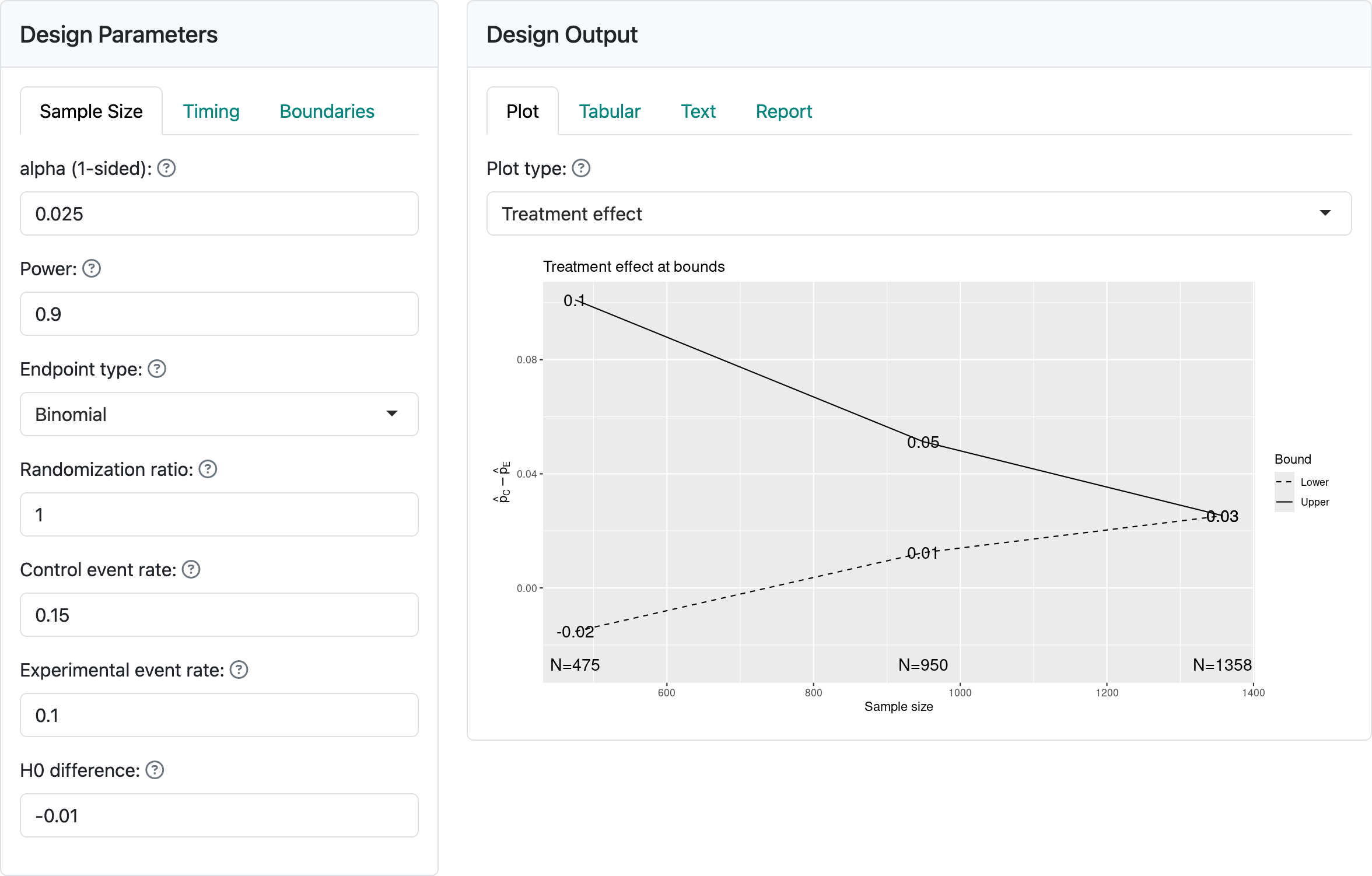### 3.1.5 Updating a binomial design at time of analysis

Select the “Update” tab and change the sample size at analyses from the continuous values to 660, 1360 and 1900. The interim analyses thus have smaller than planned sample sizes (over-running) and the final analysis has a smaller than planned sample size (under-running). Such deviations can happen if cutoffs for analysis are planned based on time rather than sample size to facilitate finalizing data or other logistics related to trial operations. You should see the following output: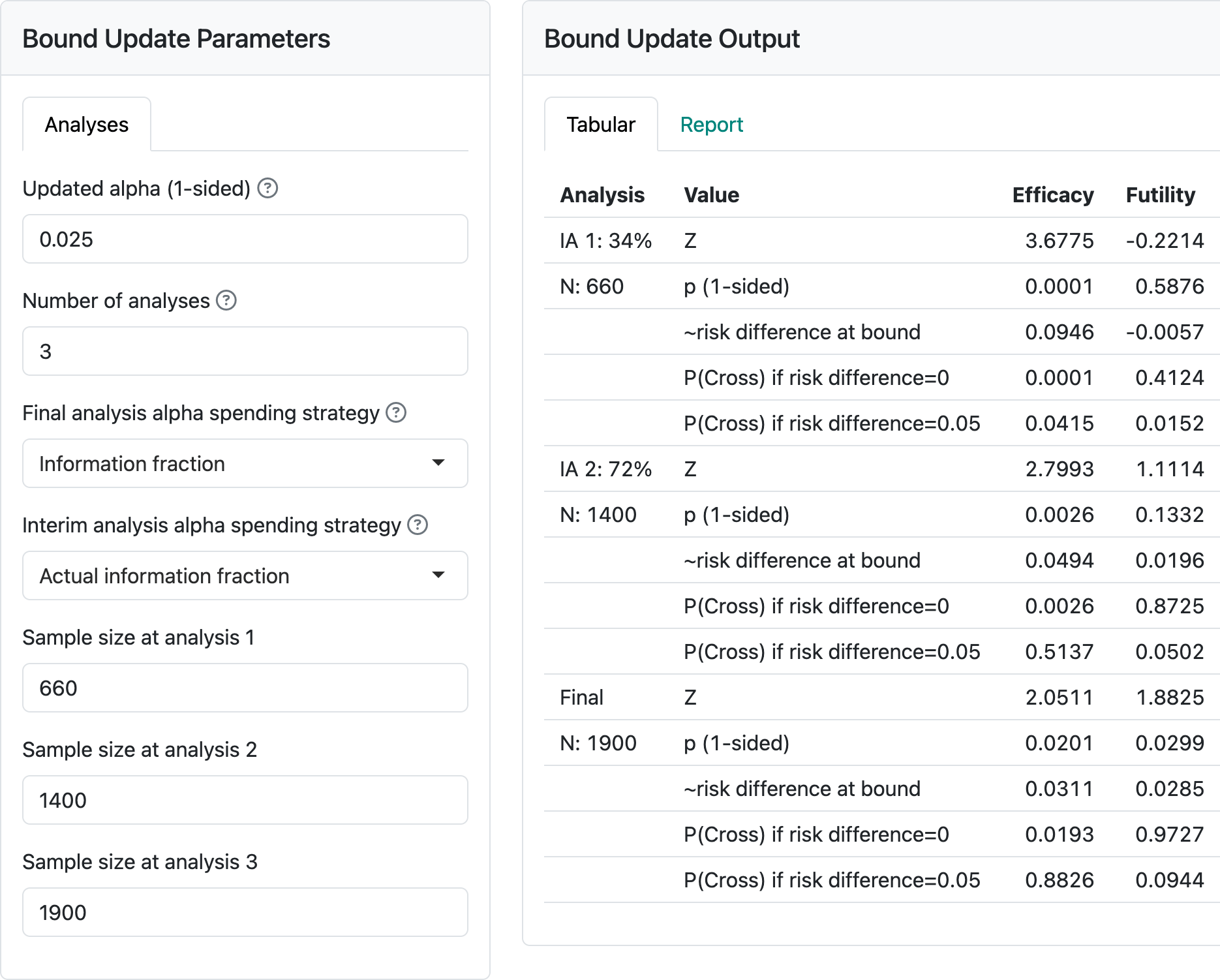Now change the controls on the left as follows:You will see that the final analysis nominal p-value cutoff changes from $$0.0211$$ to a less stringent $$p = 0.0229.$$ Now, if we further change the “Interim analysis alpha spending strategy” control to “Minimum of planned and actual information fraction”, the final nominal p-value is further relaxed to $$p=0.0235$$. Note that this is at the cost of interim p-values to cross bounds being more stringent. It would be prudent to pre-specify how spending will be performed if you may wish to apply either of the above strategies. Ensuring the full $$\alpha$$ is spent at the final analysis is particularly valuable. Changing to “Minimum of planned and actual information fraction” at interim analyses may or may not be desirable, depending on the trial under consideration.

### 3.1.6 Binomial design for response rate

We briefly consider a potential phase 2 design for response rate to illustrate:

1. Using response rate rather than failure rate for design in the interface.
2. The potential for establishing futility or early proof-of-concept with aggressive interim bounds.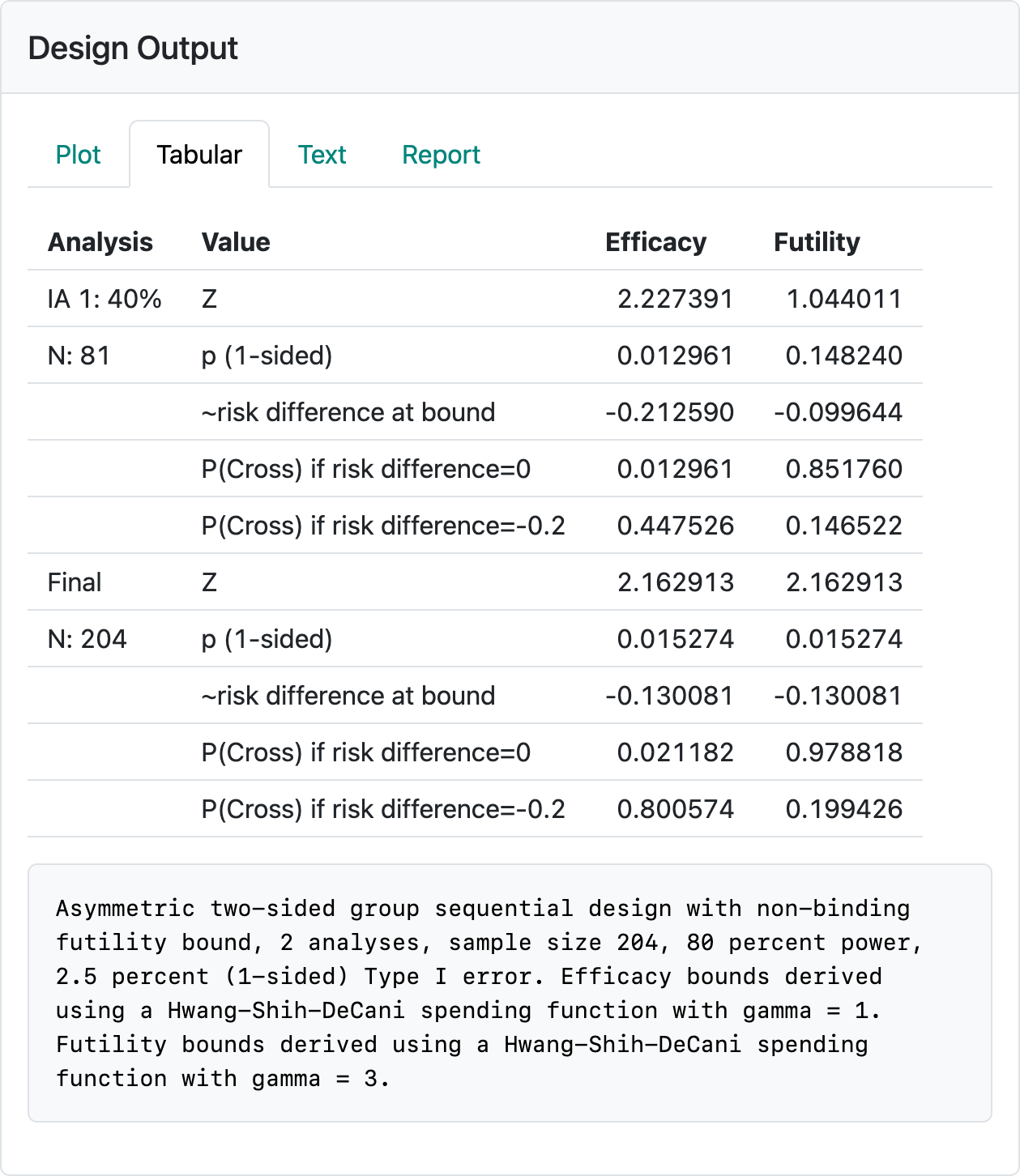The above design is saved in the file binomial-superiority.rds. You may wish to re-create it by reading the above and seeing if you can reproduce the design. The control and experimental response rates used were 0.15 and 0.35, respectively. The efficacy bounds approximate an aggressive Pocock bound (Pocock10) that would normally not be used in a Phase 3 design, but may be useful for demonstrating an early proof-of-concept in a Phase 2 study. In this case, a pause in enrollment or limiting enrollment until the interim has been performed may be a way to limit early investment in the study. The interim efficacy bound establishing efficacy (i.e., proof-of-concept to finish the Phase 2 trial) with a ~10% improvement in response rate or establishing an early go to Phase 3 with a ~20% improvement in response rate can enable early decisions to 1) stop further investment (cross futility bound), 2) accelerate further investment (cross efficacy bound), or 3) finish Phase 2 prior to further investment (cross neither efficacy nor futility bound). If you check in the “Text” tab you will note that a fixed design with no interim and the same power and Type I error can be achieved with a sample size of $$N=146$$. All of this is meant as food for thought when you design a trial; final selection of a design will be based on many considerations.

## 3.2 Normal endpoints

### 3.2.1 Normal outcomes

Normal endpoints refers to outcomes where each patient has a an outcome that is distributed according to a bell-shaped normal distribution:This could be a measure such as change in cholesterol level before and after a being treated on study. While the methods here formally assume the variance is known, this should not be a problem for moderate or large sample sizes.11

### 3.2.2 Input

The next graphic shows the input values for a trial with a normal distribution for the study outcome. We have selected “Normal” for “Endpoint type.” The “alpha”, “Power” and “Randomization ratio” controls remain unchanged from the previous section. The next control specifies the difference in means for the outcome in the experimental group compared to the control group. We have selected “Superiority” to test for superiority and we have specified equal standard deviations in the two treatment groups. Try selecting unequal to see how the controls change. In this case, we select equal standard deviations with a value of 7.712443.

Examining the output panel, we have selected the “Text” tab. At the top, we see a fixed design sample size of 100. This is the same as the fixed design sample size we chose in our example with user-defined sample size. Seeing the fixed design sample size may be the primary reason for selecting the “Text” output tab. Generally, the “Text” tab has similar information seen elsewhere in a different format. If you look at other output tabs, they will provide the same output we had previously for the user-defined sample size example. This is because we have, in both cases, $$\delta = 5$$, a fixed design sample size of 100, the same power and Type I error, and the same spending functions and interim analysis timing to define interim and final analysis boundaries. That is, as long as nothing else changes, two designs based on a common fixed design sample size result in an identical group sequential design. In addition, if the treatment effect $$\delta$$ for which each design is powered is the same then the approximate treatment effects required to cross each bound will also be the same for the two designs.